# 7. Parts difference¶

In this tutorial we are going to learn to perform transformations on the pieces using the subtraction operation. This consists of removing material from one object using the shape of another. This operation is very important because it allows drilling, hollowing, cutting, etc.

1. Open the FreeCAD application and click on the icon to create a new document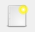.

We select the Part workbench to start designing objects in 3 dimensions.

2. At this point we are going to add the reference axes to help us place the pieces correctly.

In the menu `View... Activate or deactivate the cross of the axes.`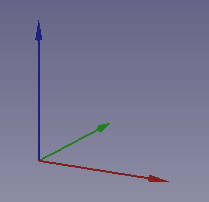3. Now we create a cube by clicking the first icon on the solid object bar.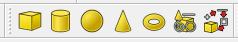and we resize the cube according to the following dimensions.

Length (Length) = 30

Width = 30

Height (Height) = 30

We select to see the piece in isometric view.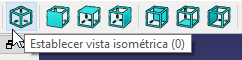4. Once the previous cube is created, we are going to subtract several small cubes from it to create a new figure.

We create a new cube by clicking on the corresponding icon.

We translate the new cube to the position x = 20, y = 0, z = 20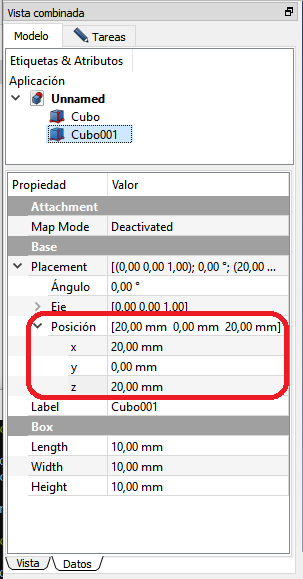With what the work screen will show us the following.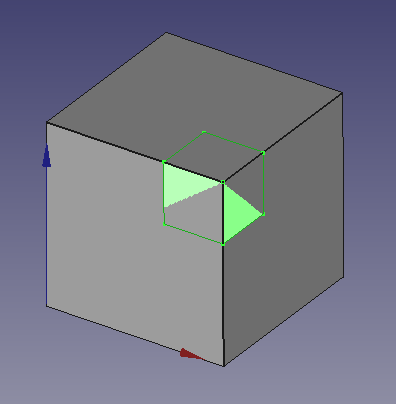5. Now let's first select the large Cube and, holding down the Control key, select the small Cube001 as well.

The order when selecting the cubes is important for the subtraction to work correctly. First the base piece is selected and then the piece to be subtracted.

Click the subtraction (Make a two-way cut) icon on the boolean toolbar.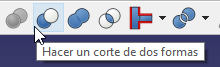The result will be like the following image.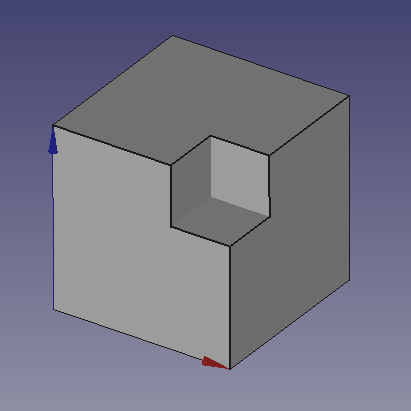6. The new piece can be used as a base piece to continue removing even more pieces.

If we create a new cube and move it to position x = 20, y = 20, z = 20

We subtract the new cube and the result will be the following.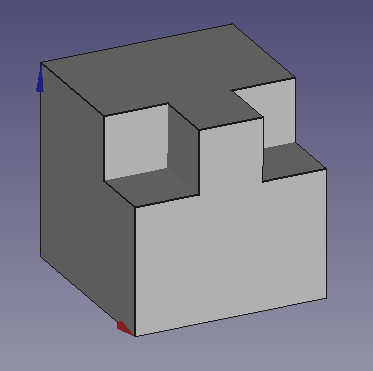## Exercises¶

1. Create a box with a side of 30mm with an interior hole of 24mm on a side, as shown in the following figure.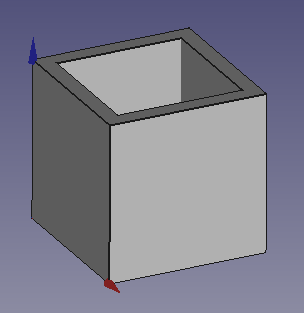Change the transparency of the outer cube to 25% so that the inner hole can be seen.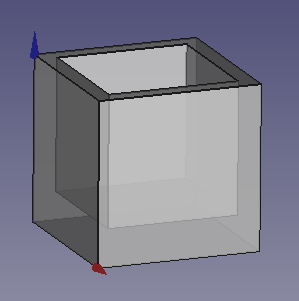2. Create a pen drive holder like the one in the figure.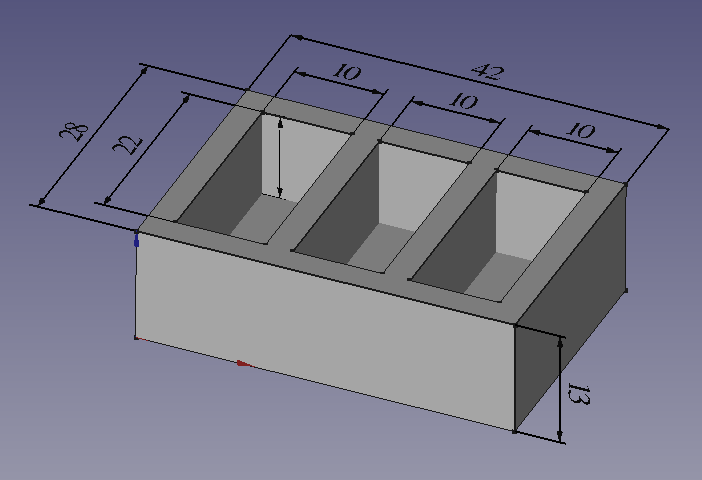## Video-tutorial¶

Making a difference.